Share this: Google+

## Finding Zeros Root of non linear equation using Excel

Suppose you want to find the root of this non-linear equation: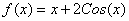The graph of the function is exhibit in the figure below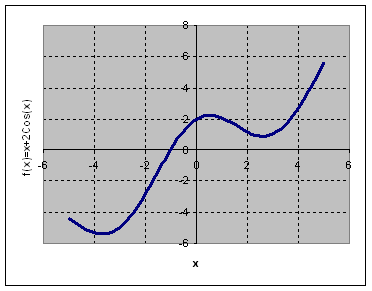From the picture above, we can guess that the root of the non-linear equation is between 0 and -2.

The steps by step procedure is as follow

1. Input the value of parameter a = 2 in cell B3. This is useful if you want to check the effect of parameter

2. Input initial value of x (you may guess any number) in cell B4

3. Type the non-linear equation in B5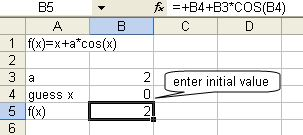4. From the menu of MS Excel, click Tools-Goal Seek and Goal Seek dialog show up.

5. Set cell B5 ( the non-linear equation ) to value 0 ( target value ) by changing cell \$B\$4 ( initial guess of x ) and click OK button.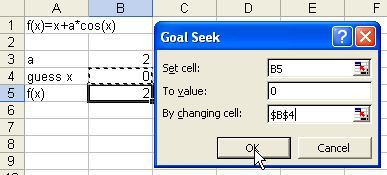6. The result is shown in the figure below. The root of the non-linear equation is -1.029. Inputting this root to the equation will make the value of f(x) = 0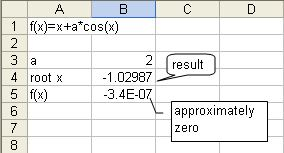Preferable reference for this tutorial is

Teknomo, Kardi (2015) Obtaining zeros of non-linear equation using Microsoft Excel. https:\\people.revoledu.com\kardi\ tutorial\Excel\ZerosNonLinearEquation.html